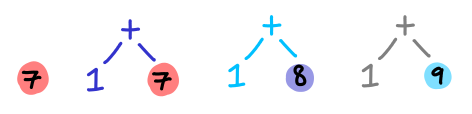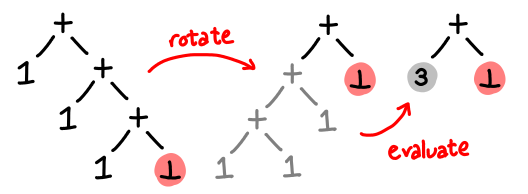the arc of software bends towards understanding

## Computing function composition

This is an addendum to my second example in Anatomy of a thunk leak, in which I’d like to propose another solution to the space leak, involving computing the composition of all of these thunks. This solution is particularly notable because it preserves the denotation of the original function, that is, that f l (undefined, undefined) = (undefined, undefined). This should be surprising, because I claimed that it would be impossible for GHC to optimize a function with that had this denotation into one without the space leak by more eagerly evaluating some thunks. There is no contradiction: the optimization we would like to apply here is one of partial evaluation. Didn’t understand that? Don’t worry, a concrete example is coming soon.

As Heinrich Apfelmus points out, the space leak can be visualized as a large graph of expressions which has not been collapsed into a single value: 1 + (1 + (1 + (1 + (1 + (1 + ...))))). We can visualize this graph being built up in successive iterations of the function:The point of introducing strictness (and thus changing the denotation of the function) is that we keep collapsing (evaluating) the tree.But notice the value highlighted in red: we must know what this value is before we can do any computation. But if this value is unknown (or, in our case, if we don’t want to evaluate it while we are forming this graph), our strategy doesn’t really work. We can’t collapse the entire tree. However, (and this is the key), because addition is associative, we can rotate the tree, and then evaluate the (now left) subtree.In effect, all of the thunks have been merged together: instead of 1 + 1 + 1 + X. we now have 3 + X. Simple! Here is the implementation:

```f l (x0, x1) = go l (0, 0)
where go [] (!c0, !c1) = (c0 + x0, c1 + x1)
go (x:xs) !c     = go xs (tick x c)

tick x (!c0, !c1) | even x    = (c0, c1 + 1)
| otherwise = (c0 + 1, c1)
```

go is essentially the strict version of f, but at the end of the iteration it returns a pair with two thunks: c0 + x0 and c1 + x1, were both c0 and c1 have been fully evaluated.

Here’s another way of thinking of how we’re doing things:It would be pretty cool if this could be done automatically, and it would pretty applicable in other domains too. Combining functions that are associative are a precious commodity when it comes to parallelization.

### 3 Responses to “Computing function composition”

1.John Salvatier says:

Is this basically a subcase of supercompilation? http://vimeo.com/15833948

2.Heinrich Apfelmus says:

Yay for graphs. :-)

I think the point is that the optimized function has no direct recursive definition. Instead, you define it in terms of a recursive helper function.

I’m not sure whether partial evaluation is relevant here. I think you can’t optimize `(1+). (1+)` to `(2+)` without knowing that `(+)` is associative. Evaluation inside the lambda doesn’t help with that.

3.Edward Z. Yang says:

John: Not sure, but IIRC supercompilation is compile time and this needs to happen at runtime.

Heinrich: Yes, that’s the straightforward translation. The correctness of this approach is ensured by the associativity of plus (though it’s probably not strictly necessary.)

Actually, the key for function composition is not the associativity of plus, but the associativity of function composition. You just need to know how to combine function appropriately.